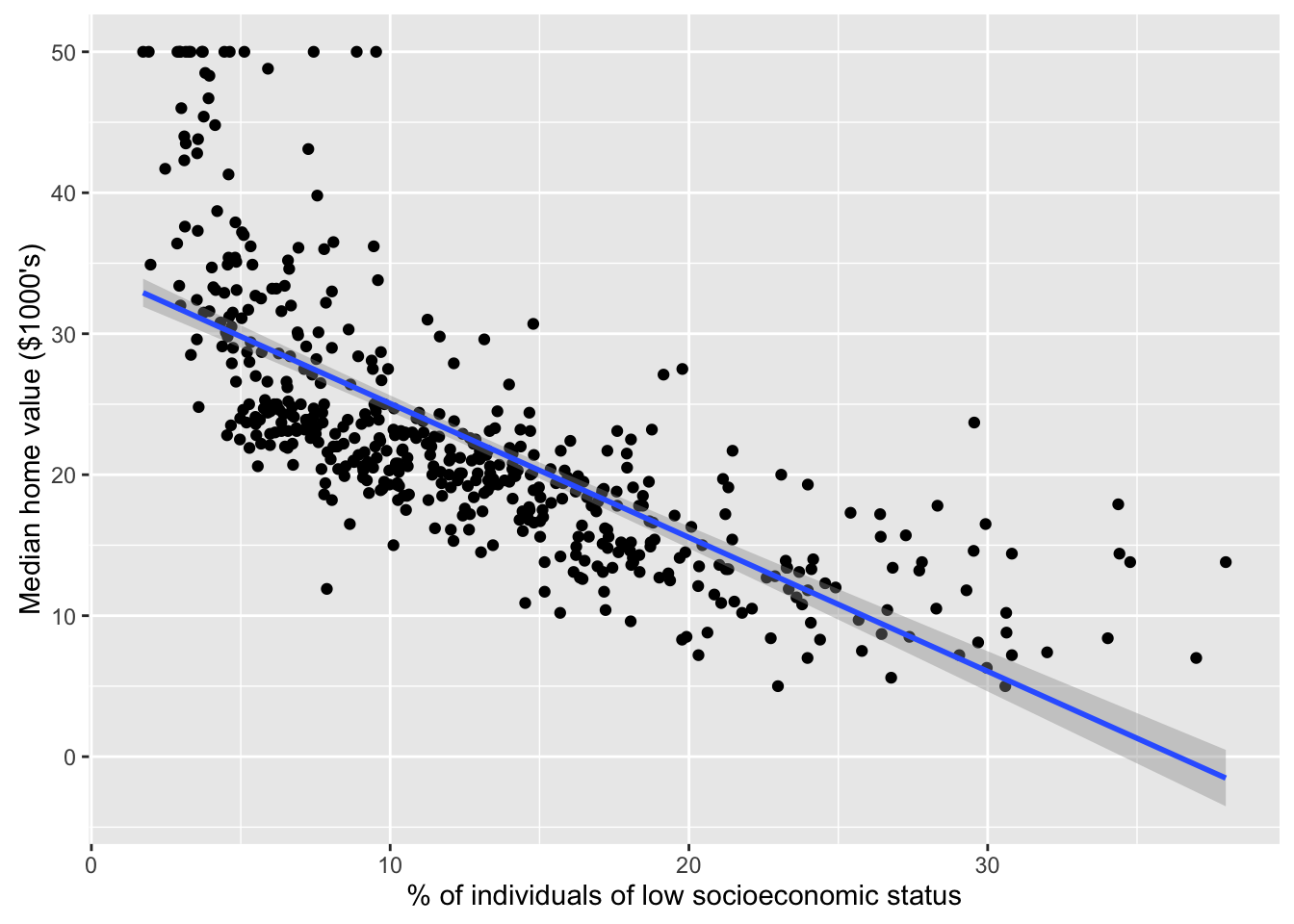### 1. Changing the author field and file name.

##### (b) Rename this file to “lab01_YourHameHere.Rmd”, where YourNameHere is changed to your own name.

Just like every other programming language you may be familiar with, R’s capabilities can be greatly extended by installing additional “packages” and “libraries”.

To install a package, use the install.packages() command. You’ll want to run the following commands to get the necessary packages for today’s lab:

install.packages("ggplot2")
install.packages("MASS")
install.packages("ISLR")
install.packages("knitr")

You only need to install packages once. Once they’re installed, you may use them by loading the libraries using the library() command. For today’s lab, you’ll want to run the following code

library(ggplot2) # graphics library
library(MASS)    # contains data sets, including Boston
library(ISLR)    # contains code and data from the textbook
library(knitr)   # contains kable() function

options(scipen = 4)  # Suppresses scientific notation

### 3. Simple Linear Regression with the Boston Housing data.

This portion of the lab gets you to carry out the Lab in §3.6 of ISLR (Pages 109 - 118). You will want to have the textbook Lab open in front you as you go through these exercises. The ISLR Lab provides much more context and explanation for what you’re doing.

Please run all of the code indicated in §3.6 of ISLR, even if I don’t explicitly ask you to do so in this document.

Note: You may want to use the View(Boston) command instead of fix(Boston).

##### (a) Use the dim() command to figure out the number of rows and columns in the Boston housing data
# View(Boston)
dim(Boston)
##  506  14
##### (b) Use the nrow() and ncol() commands to figure out the number of rows and columns in the Boston housing data.
# Edit me
nrow(Boston)
##  506
ncol(Boston)
##  14
##### (c) Use the names() command to see which variables exist in the data. Which of these variables is our response variable? What does this response variable refer to? How many input variables do we have?
# Edit me
names(Boston)
##   "crim"    "zn"      "indus"   "chas"    "nox"     "rm"      "age"
##   "dis"     "rad"     "tax"     "ptratio" "black"   "lstat"   "medv"
• The response variable is medv, which represents the median house value for various neighbourhoods in Boston.

• There are a total of 14 columns in the data. One of these is the response variable medv, which leaves 13 “predictor” or “input” variables.

##### (d) Use the lm() function to a fit linear regression of medv on lstat. Save the output of your linear regression in a variable called lm.fit.
# Edit me
lm.fit <- lm(medv ~ lstat, data = Boston)
##### (e) Use the summary() command on your lm.fit object to get a print-out of your regression results
# Edit me
summary(lm.fit)
##
## Call:
## lm(formula = medv ~ lstat, data = Boston)
##
## Residuals:
##     Min      1Q  Median      3Q     Max
## -15.168  -3.990  -1.318   2.034  24.500
##
## Coefficients:
##             Estimate Std. Error t value Pr(>|t|)
## (Intercept) 34.55384    0.56263   61.41   <2e-16 ***
## lstat       -0.95005    0.03873  -24.53   <2e-16 ***
## ---
## Signif. codes:  0 '***' 0.001 '**' 0.01 '*' 0.05 '.' 0.1 ' ' 1
##
## Residual standard error: 6.216 on 504 degrees of freedom
## Multiple R-squared:  0.5441, Adjusted R-squared:  0.5432
## F-statistic: 601.6 on 1 and 504 DF,  p-value: < 2.2e-16
##### (f) Uncomment the line below to get a ‘nice’ printout of the coefficients table
kable(coef(summary(lm.fit)), digits = c(4, 5, 2, 4))
Estimate Std. Error t value Pr(>|t|)
(Intercept) 34.5538 0.56263 61.42 0
lstat -0.9500 0.03873 -24.53 0
##### (g) Call names() on lm.fit to explore what values this linear model object contains.
names(lm.fit)
##   "coefficients"  "residuals"     "effects"       "rank"
##   "fitted.values" "assign"        "qr"            "df.residual"
##   "xlevels"       "call"          "terms"         "model"
##### (h) Use the coef() function to get the estimated coefficients. What is the estimated Intercept? What is the coefficient of lstat in the model? Interpret this coefficient.
coef(lm.fit)
## (Intercept)       lstat
##  34.5538409  -0.9500494
coef(lm.fit)["(Intercept)"]
## (Intercept)
##    34.55384
coef(lm.fit)["lstat"]
##      lstat
## -0.9500494
• The intercept in the model is 34.6.

• The coefficient of lstat in the model is -0.95. This means that for each 1% increase in the % of low socioeconomic status individuals residing in the neighbourhood, median home values on average decrease by $950. ##### (i) Here’s a ggplot command that overlays a linear regression line on a scatterplot of mdev vs. lstat. Edit the xlab and ylab arguments to produce more meaningful axis labels. Does the linear model appear to fit the data well? Explain. qplot(data = Boston, x = lstat, y = medv, xlab = "% of individuals of low socioeconomic status", ylab = "Median home value ($1000's)") + stat_smooth(method = "lm")• The linear model appears to be a pretty good fit to the data in the lstat range of 10 - 25. However, the overall relationship between median home value and the % of low socioeconomic status individuals in the neighbourhood appears to be overall non-linear.

• Here’s a plot showing a local regression fit to the data. The local regression model appears to do a better job of capturing the trends in the data.

qplot(data = Boston, x = lstat, y = medv,
ylab = "Median home value (\$1000's)",
xlab = "% of individuals of low socioeconomic status") +
stat_smooth(method = "loess")